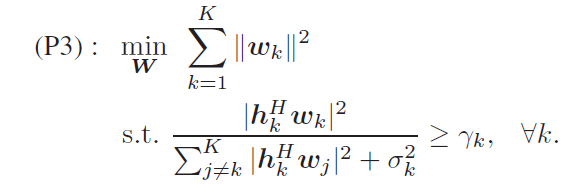# Why CVX gives Infeasible when I can prove the constraints are feasible?

I am solving a SOCP problem via SDP, but CVX keeps saying that the constraints are infeasible. However, I can prove that it is feasible because I have a closed form answer that can satisfy all the constraints.
The problem is formulated aswhere w_k are the columns of a matrix W, h_k columns of a matrix H. The only variable is W, while H, sigma_k and gamma_k are all given parameters.
The problem can be written in the form of a SDP problem:

where T is the matrix W in the previous picture. This is a problem of finding the scheme with least energy to satisfy the SINR requirements.

Apparently W = a·inv(H) can satisfy all the constraints as H·W = a·I, if a is large enough. But CVX outputs INFEASIBLE when gamma_k grows larger than zero. Does anyone knows why this occurs? I have been confused for several days and I would appreciate it if anyone can help.

My code is as follows.

``````clc;
K = 4;
M = 4;
H = (randn(K, M) + 1i.*randn(K, M));
noiseVar = 10^(-11);
gamma = ones(K, 1) .* 10;

cvx_begin sdp
variable W(M, K)
variable p
minimize(p)
bfpower = norm(W(:));
R = H * W;

subject to
bfpower <= p;
for k = 1:K
side = [R(k, :)'; sqrt(noiseVar)];
mat = [sqrt(1 + 1/gamma(k)) * (R(k, k)), side'; side, sqrt(1 + 1/gamma(k)) * real(R(k, k)) .* eye(K + 1, K + 1)];
mat == hermitian_semidefinite(K + 1 + 1);
end
cvx_end

display(W)
display(p)

``````

You haven’t provided a reproducible problem, including all input data. Therefore, readers can’t check for themselves.

You have also not shown the solver and CVX output, which might help readers assess the situation.

I found your discussion of the feasible solution a little confusing. Have you done the following? Outside CVX, assign `p` and `W` to the numerical values you claim are feasible, as done in “normal” MATLAB (W=[2 1;1 3]) Numerically evaluate `bfpower` and `mat` using those values of `p` and `W`. Are the constraints satisfied to within some numerical tolerance (for example, `mat` is symmetric and all its eigenvalues are >= -1e-8)?

Does your problem have bad scaling (very small or large magnitude non-zero numbers)?

If matters are still not resolved, follow the guidance in https://yalmip.github.io/debugginginfeasible/ , except for section 1 which does not apply to CVX.

Do you intend for `W` to be complex? If so, you st must declare it so
`variable W(M, K) complex`

Sorry for the incomplete code. I have edited so that the code can be run directly.

I was just following the guidance when I see your second reply. Yeah I want W to be complex.
I have just added the ‘complex’ into my code and it does work. Thank you so much as I am new to CVX and I would not notice this detail without your hint.

However, I sometimes encounter NAN when I keep increase the value of gamma. Is it because of the precision of calculation?

When `gamma` becomes too large, the optimization problem becomes primal ill-posed (solver log will show as dual ill-posed, because CVX provides the dual of the original problem to the solver), so the solver may not succeed in solving it as an optimization problem. That is probably symptomatic of an opitimization problem which doesn’t make much sense. However, if the objective function is removed, the problem is easily solved as a feasibility problem.

Ok I got it. Btw I find that CVX sometimes gives solutions that do not satisfy the given constraints, which happens when the entries of matrices in constraints are significantly small. And after I scale up the matrices, CVX gives optimal solutions that meets all the constraints. But I don’t think this is a ill-posed situation because scaling up the constraints actually makes no difference to the solutions. How could I avoid such situation?

Scaling matters. Solver performance is not invariant to scaling of input data. Try to keep non-zero input data to within a small number of orders of magnitude of 1. When you don’t, bad things can happen. That is reality, whether you like it or not.

Understood. Thank you very much for your time and patience.Hi zieac,
I want to solve similar problem, and I used feasibility method. However, when I implemented it, although CVX shows the problem solved, but there is no improvement in the main objective function value. I am new to CVX. I might be missing something.
I implemented your code on MATLAB, but it was showing error as shown below:

Array indices must be positive
integers or logical values.

Error in cvx_extract (line 344)
tmpv =
sum(dbcA(temp,:)~=0,2)==1;

Error in cvx_solve (line 26)
[ At, cones, sgn, Q, P, exps,
dualized ] = cvx_extract(
shim.config, shim.name );

Error in cvx_finish

Error in cvx_end (line 11)
evalin( ‘caller’, ‘cvx_finish’
);

Can you please send me the updated code at saumyac@iiitd.ac.in
That will be really helpful for me. Also, if you have some idea how to solve it using feasibility method, that would be great. Please contact me in case of any queries.

Sir, can you please tell me the CVX code of how it can be solved as a feasibility problem when the objective function is removed. I tried implementing it in the CVX, at the end it says that the problem is solved but there is no improvement after optimization. I am new to CVX, and might be missing something.